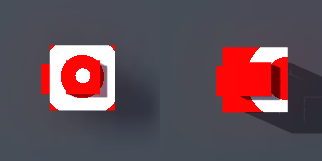This next section will explain how to alter a mesh’s texture based on internal game data, such as the gameobject’s world position.

In order to implement the desired effect first the properties code block must be expanded to include a few new items. The Center property will define the world position vector that the shader will alter itself, and the Radius property which will determine how large the effect will be.

The next alteration will be in the input struct, an extra field worldPos is added to access the mesh world position at runtime. Then we declare the new properties into the shader above the shader surf function.

The surf function’s parameters remain unchanged since the Input struct was all that needed to be altered. Inside the function some math is used to determine when to change the mesh. First the distance between the Center and worldPos vectors is stored followed by a delta calculation between 1.0 and 0.0 involving that saturate function, which is a fancy min max function.

Next the delta variable is altered using a series of step functions, which checks to see if the first parameter is larger than the second, essentially this is a fancy test to check if delta is larger than 0.3 and 0.25 is smaller than delta. This trick is used because shaders are more efficient at math than at doing branches, so while you can write simple if else statements you can achieve higher frame rates relying on pure math.Finally the mesh’s o.Albedo is assigned again using tex2D with its result scaled by the delta variable which sets the color to white. The end result of this shader is that a white circle, with a radius defined by the Radius property, will be projected onto a mesh when that mesh is moved to the position defined by the Center property.

```Shader "Custom/ShaderExperiment2" {
Properties {
_MainTex ("Texture", 2D) = "white" {}
_Center ("Center", Vector) = (0,0,0,0)
}
Tags { "RenderType" = "Opaque" }
CGPROGRAM
#pragma surface surf Lambert
struct Input {
float2 uv_MainTex;
float3 worldPos;
};
sampler2D _MainTex;
float3 _Center;